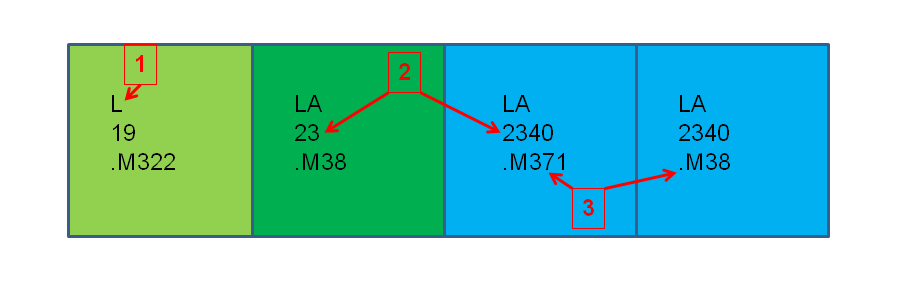# Books@UWM

A guide to finding and using eBooks from the UWM Libraries collections.

## Search@UW

#### Find articles, UW books, digital collections function searchPrimo() { document.getElementById("primoQuery").value = "any,contains," + document.getElementById("primoQueryTemp").value.replace(/[,]/g, " "); document.forms["searchForm"].submit(); } document.write( '<form id=\"primo-ve-search\" name=\"searchForm\" method=\"get\" action=\"https://wisconsin-uwm.primo.exlibrisgroup.com/discovery/search\" onsubmit=\"searchPrimo()\" enctype=\"application/x-www-form-urlencoded; charset=utf-8\">\n' ); document.write( '<input type=\"hidden\" name=\"vid\" value=\"01UWI_ML:MIL\" />\n' ); document.write( '<input type=\"hidden\" name=\"search_scope\" value=\"DN_and_CI\" />\n' ); document.write( '<input type=\"hidden\" name=\"mode\" value=\"basic\" />\n' ); document.write( '<input type=\"hidden\" name=\"lang\" value=\"en\">\n' ); document.write( '<input type=\"hidden\" name=\"tab\" value=\"MAIN\">\n' ); document.write( '<input type=\"hidden\" id=\"primoQuery\" name=\"query\" /> \n' ); document.write( '<input id=\"primoQueryTemp\" type=\"text\" placeholder=\"Search@UW - Start your search here\" value=\"\" size=\"40\"/> \n' ); document.write( '<input type=\"submit\" value=\"Go\" id=\"go\" onclick=\"searchPrimo()\" /> \n' ); document.write( '</form>' );

Use Search@UW to find books in the UWM Libraries print and online collections. You will also find books held by other UW System Libraries. Sign-in when you begin using Search@UW so that you can make requests including requesting books from other UW Schools.

How do I find a call number on the shelf?

Follow these steps when you're looking for a book on the shelf.

1. Find the correct letter combination. L comes before LA on the shelves.
2. Look for the number - be sure to look for the whole number. 19 comes before 23 and 23 comes before 2340 (two thousand three hundred forty).
3. In the last line, first locate the letter and then the decimal. .M371 will come before .M38